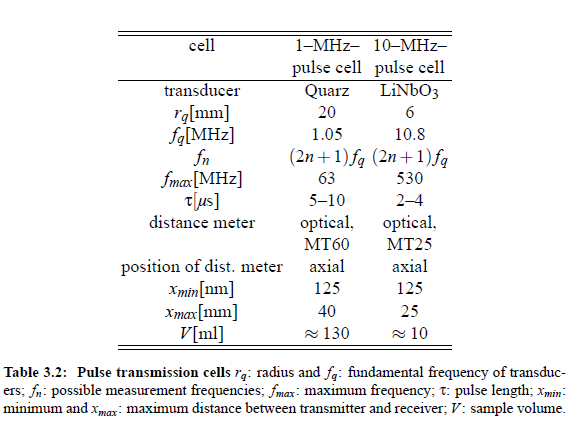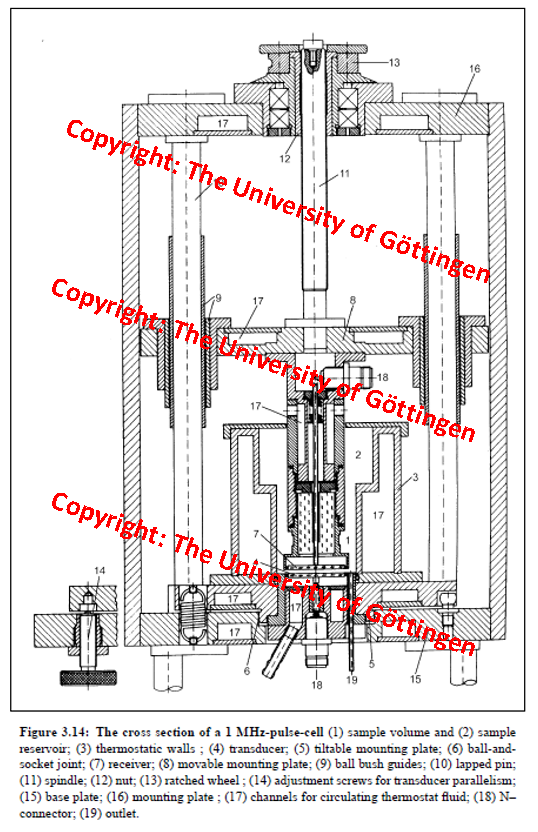Category: Projects

### Pulse-modulated traveling wave methods: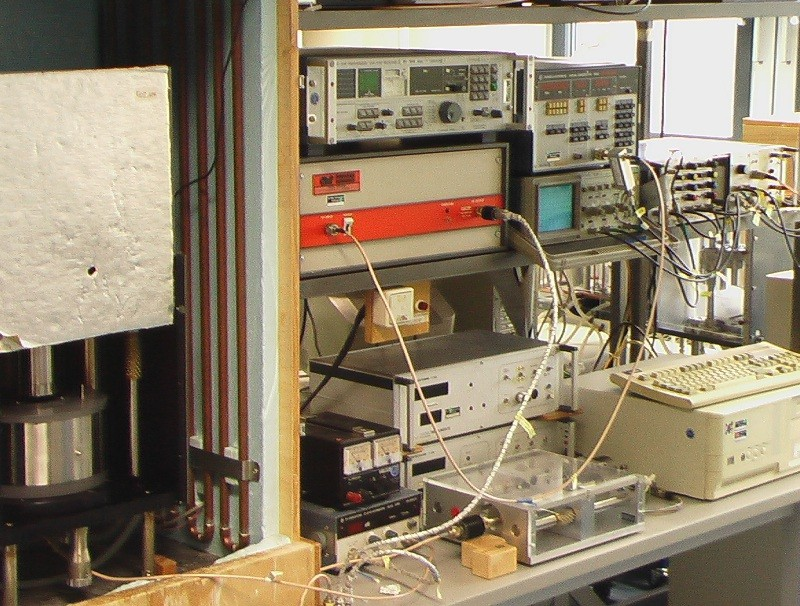At frequencies above 3 MHz the distortion of resonator curves by higher order modes may be so strong that the separation of the satellite peaks from the main resonance curve becomes impossible. However, the simple proportionality, given by the expression: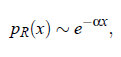allows to measure quasi-directly the sound attenuation coefﬁcient α. Pulse-modulated measurement are performed at the transducer fundamental frequency and its odd overtones which follow the (2n + 1) ·f T -law. The shiftable receiver, as shown in Fig.(3.10), at the position X 1 detects the acoustical signal and transfers this into an alternating voltage U_R( X_1). At the position X_2   the alternating voltage U_R  (X_2) is received. Hence, the attenuation coefﬁcient α follows as: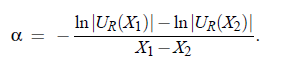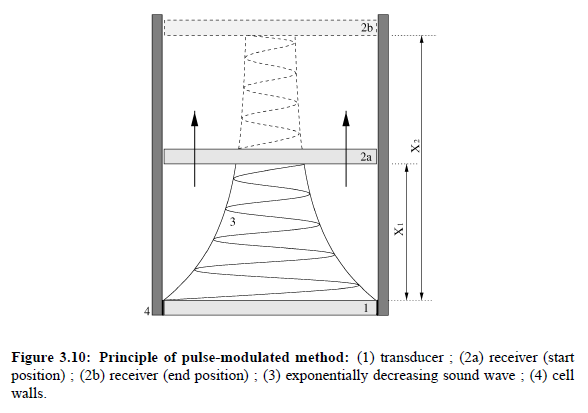Pulse  modulation  of  the  sound  wave  avoids  overlaps  between  the  original  signal  and multiply reﬂected signals as well as the electrical crosstalk. At small transducer spacing, so-called  acoustic  delay  lines  (fused  quartz)  are  used  for  pulse  separation. The  pressure amplitude p_ R  of the sound wave (taking into account multiple reﬂections) can be expressed as: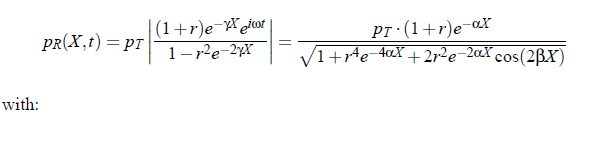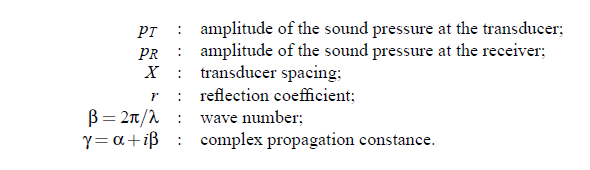The cosine function of the transfer function describes the so-called λ/2-ripple, caused by the multiple reﬂections of the signal at the transducers. For αλ > 3 the denominator of previous equation equals approximately 1, so that an exponential decay of the sound wave p R  ∼ e−αX follows. In Fig.(3.11) a plot is given as an example. It illustrates that at decreasing sample length X  the standing wave contributions of the ultrasonic ﬁeld within the cell become more and more important.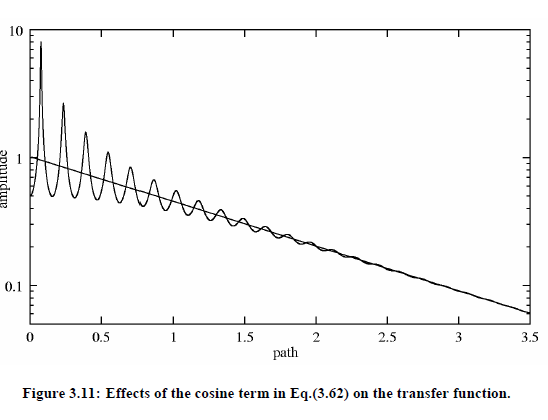fitting at a given frequency f; to experimental data yields the absorption coefﬁcient α and the sound velocity cs  = λf of the sample liquid. To raise the accuracy of α measurements it is usual to reduce the inﬂuence of the cosine function.          This can be realized with the help of the pulse modulation as mentioned before. The transducer signal is pulsed with the aid of square-wave pulse, with the pulse length following the relation:τ = 2 − 10 µs. From this relation it is evident that the knowledge of the sound velocity cs of the liquid sample is essential for correct pulse adjustments.

Sound velocity measurements:

The transducer and the receiver have to be brought to a distance fulﬁlling X  < τc/2 or X  < 3/α.  At  the  range  of  20 · λ,  the  transfer  function  is  measured  and  the  experimental data at constant frequency are ﬁtted. Finally, with the      help of cs  = λ ·f , the sound velocity cs  can be calculated.

Characteristic curve of electronic setup:

The accuracy of pulse methods depends on the accuracy of determining the characteristic curve of the electronic equipment.  In the measurement mode the signal  passes some electronic devices. At ﬁrst, the voltage U_R  has to be demodulated and ampliﬁed. Unfortunately, non-linear effects in the electronic circuit, especially in the ampliﬁer exist. Hence, the voltage U_R is subject to the characteristic curve of the receiver C (U_R) and the sound pressure amplitude at the receiver quartz: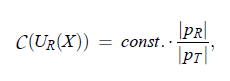Finally, it is possible, with the aid of linear regression to calculate the attenuation coefﬁcient as given: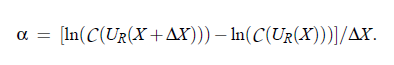Here ∆X is the measurement distance. A central role in the determination of the characteristic curve of the electronic equipment plays the cut-off piston attenuator. Calibration of the apparatus is performed after each run by switching from the measuring branch to the reference branch and utilizing the cut-off piston attenuator to vary the receiver voltage.The voltage characteristic obtained by this calibration procedure allows the correction of the originally measured U_R values.

Electronic equipment and measuring procedure: A block diagram of the electronic apparatus is shown in Fig.(3.12).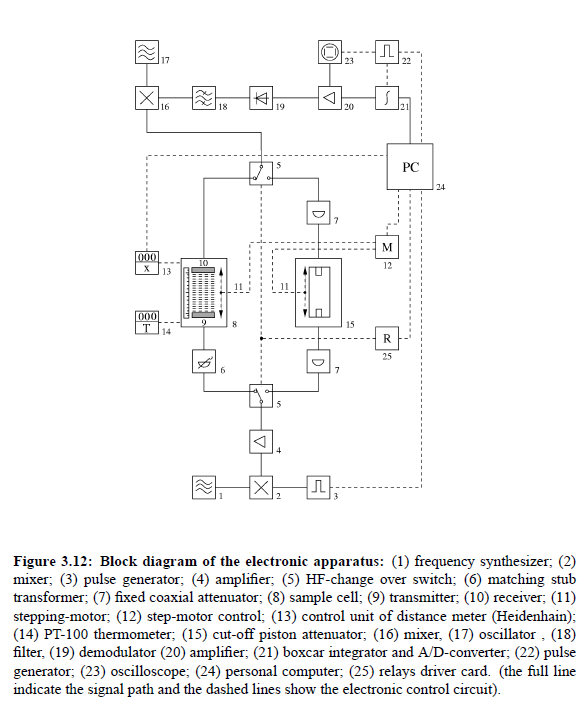The full lines ndicate the signal path and the dashed lines show the electronic control circuit. The  frequency  synthesizer  (1)  along  with  the  pulse  generator  (3)  and  the  mixer (2) generates a pulse-modulated HF signal with frequency of measurement f. The signal, via two coaxial HF-switches, is passed either through the measuring branch or the reference branch of the comparator circuit. After passing the sample cell or  the  below-cut-off  piston  attenuator,  both  signals  are  fed  via  HF  change  over switch (5) to a superheterodyne receiver (16-20). A boxcar integrator (21) adds up the signal over a sequence of 400 pulses.  Finally, the result is transferred to a personal computer and evaluated using the data of length l, measured by the digital distance meter (11) with control unit (13). The temperature is measured with a Pt-100 thermometer (14).

Pulse cell parameters:

Some relevant data of the cells are tabulated in Table (3.2).